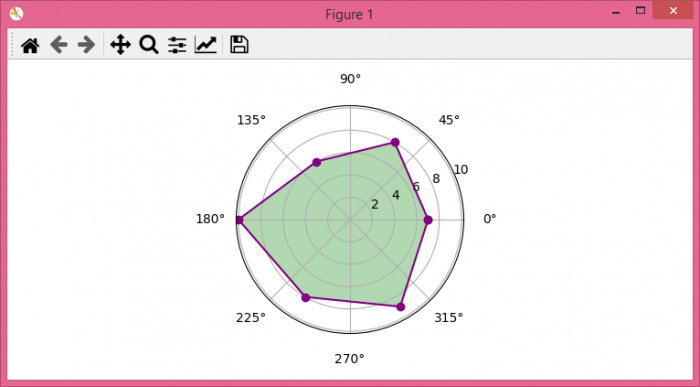# How to make a polygon radar (spider) chart in Python Matplotlib?

To make a polygon radar (spider) chart in Python, we can take the following steps −

## Steps

• Set the figure size and adjust the padding between and around the subplots.

• Create a Pandas dataframe with sports and values columns.

• Create a new figure or activate an existing figure.

• Add an 'ax' to the figure as part of a subplot arrangement.

• Based on data frame values, get the theta value.

• Get the values list of the data frame.

• Make a bar plot with theta and values data points.

• Fill the area between polygon.

• To display the figure, use show() method.

## Example

import pandas as pd
import matplotlib.pyplot as plt
import numpy as np

plt.rcParams["figure.figsize"] = [7.50, 3.50]
plt.rcParams["figure.autolayout"] = True

df = pd.DataFrame({'sports': ['Strength', 'Speed', 'Power', 'Agility', 'Endurance', 'Analytical Aptitude'], 'values': [7, 8, 6, 10, 8, 9]})

fig = plt.figure()

theta = np.arange(len(df) + 1) / float(len(df)) * 2 * np.pi

values = df['values'].values
values = np.append(values, values)

l1, = ax.plot(theta, values, color="purple", marker="o", label="Name of values")

plt.show()